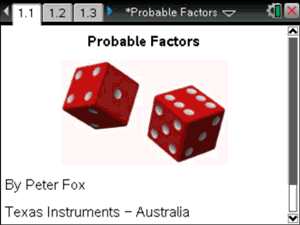# Activities

•• ##### Subject Area

• Standard: National Curriculum 7-10: Number and Algebra: Patterns and algebra
• Standard: National Curriculum 7-10: Number and Algebra: Linear and non-Linear Relationships
• Standard: National Curriculum 7-10: Statistics and Probability: Chance
• Standard: VCE: Mathematical Methods: Mathematical Methods

• ##### AuthorMiddle
Aust Senior

120 Minutes

• ##### Device
• TI-Nspire™ CAS
• ##### Software

TI-Nspire™ CAS

3.2

## Probable Factors#### Activity Overview

What is the probability that a randomly generated quadratic will factorise? This investigation looks at a significantly reduced set of quadratics using dice to generate the coefficients. The coefficient of x is the sum of two dice and the constant is the product of two dice. Simulation is used for predicting the probability followed by theoretical computations.

#### Objectives

• Apply the distributive law to the expansion of algebraic expressions, including binomials, and collect like terms where appropriate (ACMNA213)
• Expand binomial products and factorise monic quadratic expressions using a variety of strategies (ACMNA233)
• Explore the connection between algebraic and graphical representations of relations such as simple quadratics, circles and exponentials using digital technology as appropriate (ACMNA239)
• Describe the results of two- and three-step chance experiments, both with and without replacements, assign probabilities to outcomes and determine probabilities of events. Investigate the concept of independence (ACMSP246)